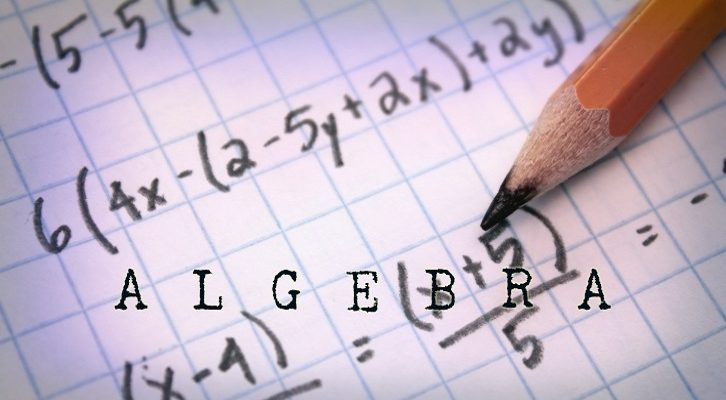# CAT – Questions on Inequalities

Inequalities has become a more important topic to focus on in the past few years. I have Few questions on inequalities.

Questions:

1. Sum of three distinct natural numbers is 25. What is the maximum value of their product?

2. x ( x + 3) ( x + 5) (x + 8) < 250. How many integer values can x take?

Question 1: 560
Question 2: 11 values

Qn 1. Sum of three distinct natural numbers is 25. What is the maximum value of their product?

Let the three natural numbers be a, b and c. We know that a + b + c = 25.

Let us substitute some values and see where this is headed
a = 1, b = 2 c = 22, Product = 44
a = 2, b = 3 c = 20, product = 120
a = 5, b = 6 c = 14, product = 420

The numbers should be as close to each other as possible. (Just trial and error and one can figure this out). This property is an extension of the AM-GM Inequality.

25/3 ~ 8.  25 divided by 3 is approximately equal to 8. So, we should choose a, b, and c close enough to 8

8 + 8 + 9  = 25. But the numbers need to be distinct. 8 * 7 * 10 come closest. The maximum product would be 560.

Qn 2. x ( x + 3) ( x + 5) (x + 8) < 250. How many integer values can x take?

Straight away we can see that x = 0 works. The product goes to zero in this case. When x takes values -3, -5 or -8, the product will go to zero and the inequality holds good.

Now, let us substitute some other values
When x = 1 => Product = 1 * 4 * 6 * 9 = 216 < 250 So, x =1 holds good
When x = 2, the product is clearly greater than 250.

So, thus far, we have seen that for x = 0 , -3 -5 -8 or 1;  the above inequality holds good.

There are 4 terms in this product. If all 4 are positive or all 4 are negative, the product will be positive. If exactly one term is positive or exactly one term is negative, the product will be negative.

Whenever the product is negative, the inequality will hold good. So, let us find the values of x for which the product will be negative

x = -1 or -2, the product is negative, so the inequality will hold good

Let us think of other values of x for which the product is negative. For the product to be negative, either 1 or 3 of the four terms should be negative.

When x is -6 or -7, three of the terms are negative and the product is negative.

So, for x = 0, 1, -3, -5, -8, -1, -2, -6 or -7 this holds good. We have seen 9 values thus far.

In ascending order, the values are -8, -7, -6, -5, -3, -2, -1, 0, 1

The value in between that we have thus far not verified is -4. Let us try -4 as well. In this case the product is -4 * -1 * 1 * 7 < 250

For x = -9, product is -9* -6 * -4 * 1 = 216 < 250

Clearly for x = -10 or lesser the inequality does not hold good.

So, the inequality holds good for 11 terms.  x can take all integers from -9 to +1 (both inclusive).